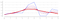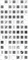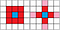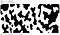# Of umbrellas, transducers, reactive streams & mushrooms (Pt. 3)

1D & 2D convolutions, functional, branchless, cellular automata

This is part 3 of an ongoing series introducing & discussing projects of the thi.ng/umbrella monorepo. Other parts:

• Part 1 — Project & series overview
• Part 2 — HOFs, Transducers, Reducers, ES6 iterables
• Part 4 — Disjoint Sets, Graph analysis, Signed Distance Fields

Having covered some transducer basics and related functional programming concepts in the previous post, we are now ready to apply some of that knowledge to start creating the world for our game.

## A convoluted approach to Cellular Automata

Theoretically, a convolution involves computing the integral over the infinite interval -∞ .. +∞. However, for our purposes, we can restrict our two functions to a finite domain and a discreet one to boot. In practice this means, rather than having to compute the integrals, we can work with and express our functions as fixed size arrays. And this itself has the following added benefits:

• we can store arbitrary (numeric) data in our arrays without needing to repeatedly re-evaluate an actual, possibly complex or even unknown function
• we can express a single convolution/integration step (i.e. of a single sliding window of function f(x)) as a dot product of two vectors.

The dot product is simply the sum of the component-wise products of two vectors:

`const dot3 = (a, b) =>  a * b + a * b + a * b;// or more generally (for any vector length)const dot = (a, b) =>  a.reduce((dp, a, i) => dp + a * b[i], 0);dot([1,2,3], [10,20,30])// 140 = 1 * 10 + 2 * 20 + 3 * 30`

As an aside: The dot product is one of these magic little gems I find myself running into in all sorts of domains, and not just in geometry related fields. Indirectly, on a fundamental level, most of NN-based machine learning is a long, nested sequence of dot products (via matrix multiplications)… Hence also the advantage of using GPUs for ML, which are hardware optimized for this computation.

Let’s start with a simple 1D convolution process, built via transducers. First, we need to define some (random) test data:

`// signalconst signal = [1, 2, 2, 2, 8, 10, 0, 0, 5, 4];// convolution coeffs / weightsconst kernel = [0.25, 0.5, 0.25];`

The next thing we require is a sliding window of all `signal` values. This sliding window should always be the same size as `kernel` — in order to compute the dot product, both vectors must be the same size. If you paid attention in the previous article, we’ve already introduced the `partition` transducer for that precise effect, i.e. segmenting a linear input sequence into overlapping or non-overlapping chunks of a given size.

`import * as tx from "@thi.ng/transducers";// use iterator version for brevity// make partitions same size as `kernel`// use a step size of 1 to create sliding window effect[...tx.partition(kernel.length, 1, false, signal)]// [ [ 1, 2, 2 ],//   [ 2, 2, 2 ],//   [ 2, 2, 8 ],//   [ 2, 8, 10 ],//   [ 8, 10, 0 ],//   [ 10, 0, 0 ],//   [ 0, 0, 5 ],//   [ 0, 5, 4 ] ]`

This is almost perfect, but not yet good enough! We’re only getting 8 result windows for our 10 input values. In general, with a partition size `k` and a step size of 1, `partition` will always produce `n — k + 1` outputs for `n` inputs. In order to process the very first and last value of `signal`, we also need this tuple `[0, 1, 2]` at the front and `[5, 4, 0]` at the end. The sliding window should always have the current value to be processed at the center index. One way to achieve this is via dynamically padding the input with some zeroes on either end, using the `concat` iterator:

`[...tx.concat(, [1, 2, 3], )]// [ 0, 1, 2, 3, 0 ][...tx.partition(3, 1, false, tx.concat(, signal, ))]// [ [ 0, 1, 2 ],  <- new//   [ 1, 2, 2 ],//   [ 2, 2, 2 ],//   [ 2, 2, 8 ],//   [ 2, 8, 10 ],//   [ 8, 10, 0 ],//   [ 10, 0, 0 ],//   [ 0, 0, 5 ],//   [ 0, 5, 4 ],//   [ 5, 4, 0 ] ] <- new`

Another alternative would be to configure the input `signal` in such way that we could consider both ends of the array not separate, but joined. That is, to consider the `signal` array as a piece of tape and then join the ends, forming a loop/ring. We will later use this approach in two dimensions to create a seamless, tiled terrain (and topologically speaking, a torus).

In our current 1D case, the iterator which can help us with this is called `wrap`:

`// prepend & append 1 value on either side[...tx.wrap([1, 2, 3, 4, 5], 1)]// [ 5, 1, 2, 3, 4, 5, 1 ]`

Now it’s only a small step to compute a full convolution: We do this by composing the `partition` transform with another `map` operation to compute the dot product of the sliding window with the kernel. We also add optional support for the wrap-around behavior:

`const convolve1d = (signal, kernel, wrap = false) => {  const kw = kernel.length >> 1;  return tx.transduce(    tx.comp(      tx.partition(kernel.length, 1),      tx.map((window) => dot(window, kernel))    ),    tx.push(),    wrap      ? tx.wrap(signal, kw)      : tx.concat(tx.repeat(0, kw), signal, tx.repeat(0, kw))  );};convolve1d(signal, kernel);// [ 1, 1.75, 2, 3.5, 7, 7, 2.5, 1.25, 3.5, 3.25 ]// with wrap-aroundconvolve1d(signal, kernel, true);// [ 2, 1.75, 2, 3.5, 7, 7, 2.5, 1.25, 3.5, 3.5 ]`

Some notes/observations:

• The size of the `kernel` should always be odd and usually ≥ 3. To avoid having to explicitly round off `kw`, we use bit shifting for the same effect.
• For a kernel size `k`, when wrap-around is enabled, only the first and last `floor(k/2)` values will be different compared to the non-wrap-around version. However, this is only true for a single convolution step. If the result is repeatedly convolved again, eventually all values will be impacted.
• For the non-wrap-around version, we’re using the `repeat` generator to produce the right number of zeroes, e.g. `[…repeat(0, 3)]` => `[0, 0, 0]`Iterative convolution of our original 10 test values / signal (blue) with kernel [¼, ½, ¼] , convolved results shown in reds — essentially a very basic low-pass filter.

## Iterative convolution

thi.ng/transducers provides several options for creating an iterative process (will only briefly discuss a couple).

iterate()

Classic, infinite, iterative induction, feedback: f(t+1) = f(f(t)). Because `iterate` creates an infinite sequence/generator, be sure to limit it via a suitable operator, like `take`:

`[...tx.take(4,  tx.iterate((x) => convolve1d(x, kernel), signal))]// [ [ 1, 2, 2, 2, 8, 10, 0, 0, 5, 4 ],//   [ 1, 1.75, 2, 3.5, 7, 7, 2.5, 1.25, 3.5, 3.25 ],//   [ 0.9375, 1.625, 2.3125, 4, 6.125, 5.875, 3.3125, ... ],//   [ 0.875, 1.625, 2.5625, 4.109375, 5.53125, 5.296875, ... ] ]// reduce w/ `last()` if you're only interested in final resulttx.transduce(  tx.take(4),  tx.last(),  tx.iterate((x) => convolve1d(x, kernel), signal))// [ 0.875, 1.625, 2.5625, 4.109375, 5.53125, 5.296875, ... ]`

scan()

This is a higher-order, stateful transducer, wrapping an inner reducer. It’s a generalization of the Prefix Sum operator. Since this is all quite a mouthful, let’s see it in action. Also recall `reductions` from the previous article…

`// actual "prefix sum" using `scan` w/ inner `add` reducer// then using `str` reducer to join outer resultstx.transduce(  tx.scan(tx.add()),  tx.str(" -> "),  tx.repeat(1, 4))// "1 -> 2 -> 3 -> 4"// compose 2 scanstx.transduce(  tx.comp(    tx.scan(tx.count(100)),    tx.scan(tx.pushCopy())  ),  tx.push(),  tx.repeat(0, 4))// [ , [101, 102], [101, 102, 103], [101, 102, 103, 104] ]`

Stateful here means that the results produced by `scan` are dependent on previous inputs (kind of the whole point of this operator, since it’s wrapping a reducer). There’re several other stateful transducers, e.g. `dedupe`, `distinct`, `partition`, `throttle` etc. However, none of them are using global state and their internal stateful nature is more or less an implementation detail.

To put `scan` to use for our convolution, we need to define our own custom reducer (which, if you remember from the previous article, is just a 3-element array of functions). This currently seems way more effort than using `iterate`, but `scan` being a transducer instead of a generator means it’s more flexible in terms of supporting different data flow scenarios (as we will see v. soon).

`tx.transduce(  tx.scan([    () => signal,                       // init    (acc) => acc,                       // completion    (acc, _) => convolve1d(acc, kernel) // reduction  ]),  tx.push(),  // collect all iterations in array  tx.range(4) // compute 4 iterations);// [ [ 1, 1.75, 2, 3.5, 7, 7, 2.5, 1.25, 3.5, 3.25 ],//   [ 0.9375, 1.625, 2.3125, 4, 6.125, 5.875, ... ],//   [ 0.875, 1.625, 2.5625, 4.109375, 5.53125, ... ],//   [ 0.84375, 1.671875, 2.71484375, 4.078125, ... ] ]`

## Wolfram automata

A cellular automaton consists of three pieces of data:

1. An array holding the cell state of the current generation. This is straightforward, especially for 1D automata. For our project, we are only dealing with cells with 2 states: dead or alive (`0` or `1`). This also is the most common case but by no means the only option. In other words, a “generation” in CA terms is the discrete function to be convolved, just as we’ve seen earlier, albeit with a more limited set of values.
2. A convolution kernel (another array), defining the size and shape of each cell’s neighborhood. In terms of convolution, these are the weights of neighboring cells: a weight of `1` includes that neighbor, a `0` ignores it. For 1D CAs the kernel usually includes one or more cells on either side. For 2D and higher, the neighborhood usually defines a ring (or cross) of cells around an empty center. We will get back to that later.
3. An encoding of birth & survival rules for a cell. These rules define the overall behavior, lifespan, and complexity of the resulting automata. In John Conway’s Game of Life, the most famous CA of all (and still an active area of research, even after decades), there’re only two rules: 1) A currently dead cells becomes alive if it has exactly 3 alive neighbors, 2) A live cell remains alive if it has 2 or 3 alive neighbors, else it dies.

In general, only cells selected by the kernel have an impact on the next state of the cell being processed. The beauty of CAs is that very simple behaviors on the local level of a few individual cells can lead to highly complex global behaviors of the entire simulated CA universe.

Another thing to keep in mind (if you’re of a philosophical nature): The entire evolution of the CA is completely defined by the state of the first generation and the rules… This is Predeterminism in action.

After lots of experimental research throughout the 1950-70s (Barricelli, Wiener, Hedlund, Conway etc.), only in the early 80s, Stephen Wolfram was the first to systematically explore and document properties and behaviors of cellular automata, primarily the binary-state, 1D versions. He later summarized this work in the 1200 page tome A New Kind of Science.Visualizations of a selection of the possible 256 rules of Wolfram automata

In the classic configuration, there’re 256 possible Wolfram automata. The “classic” refers to a kernel size of 3 cells (with equal weight) and therefore selecting the current cell plus a neighbor cell on either side. With this configuration, there’re 8 (2³) possible outcomes for each cell, depending on the current pattern selected by the kernel. Here’re the state transitions for Rule 110, considered a universal, elementary automaton:

The first row shows all 8 possible cell states for a given kernel and the 2nd row a rule specific outcome for each state. If you’re familiar with binary notation, you should recognize the bit pattern in the last row represents 110 (decimal):

110 = 0*128 + 1*64 + 1*32 + 0*16 + 1*8 + 1*4 + 1*2 + 0*1

110 = 64 + 32 + 8 + 4 + 2

## Into the land of bits

`import { bits } from "@thi.ng/transducers-binary";// expand each input number into 8 bits[...bits(8, )]// [ 0, 1, 1, 0, 1, 1, 1, 0 ] -> same as in above table`

By default `bits` will produce a bit sequence starting with the most-significant bit of each input value, but we can also invert the order by starting with the least significant bit:

`const rule = [...bits(8, false, )]// [ 0, 1, 1, 1, 0, 1, 1, 0 ]`

This is more useful for our purposes since we will use the resulting bit array as a lookup table to apply the rule behavior to each cell in a completely branchless manner. You’ll find most other CA tutorials employ all sorts of conditional constructs to determine the new state of a cell. Here, we’ll have none of that and instead solve this task via some binary trickery, not just in the current 1D case, but also later in 2D. Another huge benefit of that approach is that our CA is now completely data-driven and no code changes have to be performed if we’re changing the rule(s).

With `rule` encoded as an array in LSB order, we can now change our kernel weights to include position info (relative to the kernel) of each cell. Since we’re in the binary domain, we assign each kernel value a different power of 2:

`// left cell = 1// center cell = 2// right cell = 4const kernel = [1, 2, 4];`

Now the “trick” is that if we compute the dot product of this kernel with a cell pattern from the current CA generation, the resulting number can be directly used as an index into our rule-specific lookup table to decide a cell’s fate in the next generation. In other words, calculating `dot([1,2,4], bitpattern)` provides us with the decimal/numeric version of the given bit pattern, which is then used to obtain a 0 or 1 from the `rule`.

`dot(kernel, [0,1,1])// 6rule// 1rule[dot(kernel, [1,1,1])] // 0`

Compare the results with the above rule table to verify…

The same can be done to convert an array of digits in other number bases / radixes, here using digits in natural ordering:

Decimal: `dot([100, 10, 1], [5,1,2])` => `512`

Hexadecimal: `dot([256, 16, 1], [7, 15, 14])` => `0x7fe`

## Textmode forever!

`import { comp, map, scan, step } from "@thi.ng/transducers";import { bits, randomBits } from "@thi.ng/transducers-binary";const kernel = [1, 2, 4];const rule = [...bits(8, false, )];// randomized first generationconst seed = [...randomBits(0.25, 32)];// rename convolve1d -> evolve1d// add rule applicationconst evolve1d = (signal, kernel, rule, wrap = false) => {  const kw = kernel.length >> 1;  return tx.transduce(    tx.comp(      tx.partition(kernel.length, 1),      tx.map((window) => dot(window, kernel)),      // !!!NEW!!! rule lookup / application      tx.map((x) => rule[x])    ),    tx.push(),    wrap      ? tx.wrap(signal, kw)      : tx.concat(tx.repeat(0, kw), signal, tx.repeat(0, kw))  );};// stepwise update// computes next generation & formats itconst update = tx.step(  tx.comp(    tx.scan([      () => seed,      (acc) => acc,      (acc, _) => evolve1d(acc, kernel, rule)    ]),    tx.map((gen) => gen.map((x) => " #"[x]).join("")),  ));update();'         ##   # #    ##       #  #              'update();'        #  # ## ##  #  #     ######             'update();'       #####      #######   #      #            'update();'      #     #    #       # ###    ###           'update();'     ###   ###  ###     ##    #  #   #          'update();'    #   # #   ##   #   #  #  ###### ###         'update();'   ### ## ## #  # ### #######          #        'update();'  #          ####            #        ###       '`

Adding a few bits of UI to this and allowing users to switch between two different kernel sizes, one can play with this for the next few hours:

Note: when selecting a kernel width of 5 in the above demo, the number of possible rules grows from 256 to 4 billion (32 bit numbers)! You might get lucky finding some new interesting rule / pattern by typing in large random numbers. If you do, please share the Rule ID in the comments.

Since the browser demo utilizes several other aspects (non-CA related) which we will only start covering in follow up articles, I’ve extracted the key parts of that example as non-interactive command line version. The full code is shown below. This is meant to be run in an ANSI compatible terminal, via `node`. You can cancel the script via Control+C.

Note: The demo uses convolution related functions from the thi.ng/transducers package, instead of the functions created earlier in this article. Please see the package docs for further reference and (minor) differences:

## From 1D to 2D

As mentioned earlier, a 2D convolution kernel usually defines/selects a ring of cells around an empty center cell — at least for CA purposes. In the general case, the weights can be (and often are) any numeric value. Von Neumann’s original CA kernel neighborhood is still one of the most commonly used, however, the larger Moore neighborhood is probably the most popular choice and also used by the Game of Life:Moore vs Von Neumann neighborhoods / kernels. The red cells are used to collect cell states to determine the next state of the blue cell in the center.

Instead of updating our existing 1D convolution function for 2D, we will continue to use the existing functionality of the thi.ng/transducers package, as shown in the above example code.

First, we’re building a 2D kernel of a Moore neighborhood, a 9 element array (3x3) of 1’s, with the center cell a zero:

`// 3x3 Moore neighborhoodconst moore = [  1, 1, 1,  1, 0, 1,  1, 1, 1];// weights and XY offsets of each neighbor cellconst kernel = tx.buildKernel2d(moore, 3, 3);// [ [ 1, [ -1, -1 ] ],//   [ 1, [ 0, -1 ] ],//   [ 1, [ 1, -1 ] ],//   [ 1, [ -1, 0 ] ],//   [ 0, [ 0, 0 ] ],//   [ 1, [ 1, 0 ] ],//   [ 1, [ -1, 1 ] ],//   [ 1, [ 0, 1 ] ],//   [ 1, [ 1, 1 ] ] ]`

Next up are the rules of the game: For the Game of Life (GoL), a new cell is born if it has 3 alive neighbors and remains alive if 2 or 3 alive neighbors. Like before, these rules can be encoded as a lookup table, this time with 18 values: The first 9 for currently dead cells, the other 9 for those alive:

`const rules = [  // next states for currently dead cells  0, 0, 0, 1, 0, 0, 0, 0, 0,  // for alive cells  0, 0, 1, 1, 0, 0, 0, 0, 0];`

Also as before, this table can be expressed as a single 18-bit number:

`// Game of Life = 6152[...txb.bits(18, false, )][ 0, 0, 0, 1, 0, 0, 0, 0, 0,   0, 0, 1, 1, 0, 0, 0, 0, 0 ]`

Our evolution function becomes:

`const evolve2d = (src, kernel, rules, width, height, wrap) =>  transduce(    comp(      // sum up neighbors      tx.convolve2d({ src, width, height, kernel, wrap }),      // lookup & apply rules      tx.mapIndexed((i, n) => rules[n + src[i] * 9])    ),    tx.push(),    tx.range2d(width, height)  );`

A short explanation of the `rules[n + src[i] * 9]` expression: Since both our kernel and cell-matrix only contains zeroes or ones, the convolution result for each cell is the number of alive neighbors. To determine the right rule, we also need to take into account if the cell was currently dead or alive. The `src[i] * 9` part takes care of this. The `n` argument is the number of alive neighbors.

The cellular automata example in the thi.ng/umbrella repo implements the approach described here. By switching our rules to the configuration shown below, we can create cave / tunnel-like stable structures instead of the constantly changing patterns produced by GoL. In the next article, we will use this as the basis for extracting areas walkable/reachable by the player and to extract a terrain elevation map from the generated 1-bit image, using signed distance fields.2D CA example shown with the config used to create the terrain for our game — Live demo / Source code

## The end

As always, if you’ve got any questions or feedback, please do get in touch via Twitter, the GitHub issue tracker, join our Discord or discuss on Hacker News.

Other parts:

• Part 1 — Project & series overview
• Part 2 — HOFs, Transducers, Reducers, ES6 iterables
• Part 4 — Disjoint Sets, Graph analysis, Signed Distance Fields

PS. To prove textmode can still be hip, here’re a few more demoscene productions for your viewing pleasure:

Written by